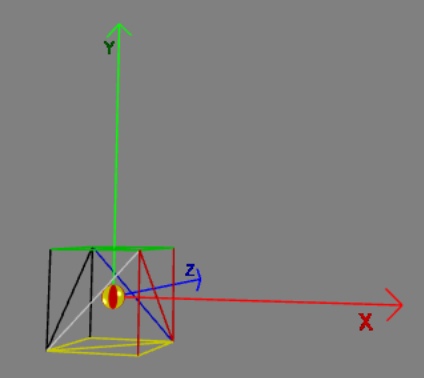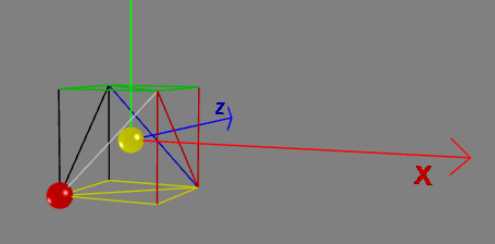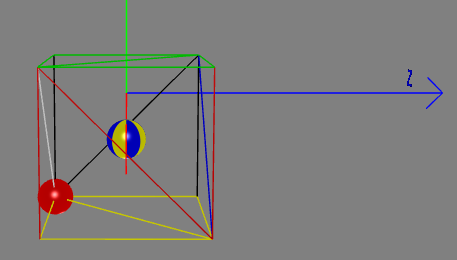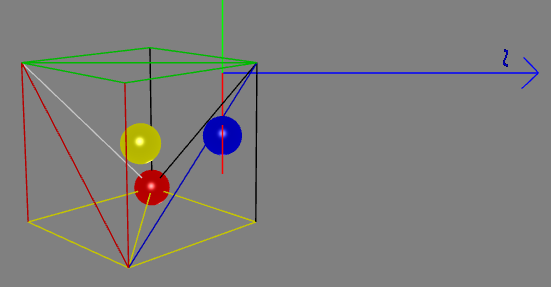# Pivots

## A Pivot

A pivot in Babylon.js is an alternative method to using a parent to set the center of transformation for a mesh, that is the point used as the center of rotation or the center of enlargement. Using setPivotPoint produces different behavior than setting a pivot point in tools such as 3DS Max and Maya and a parent should be used instead to produce a closer match to the behavior of these applications.

On this page the demonstration mesh is a cube with side length 1. When a mesh is created the pivot (red sphere) is always at the local origin (yellow sphere); the local origin and created origin are at the world space origin of (0, 0, 0). When the pivot and local origin are coincident they are shown as a red and yellow striped sphere, as in Fig 1.Fig 1 Cube with side length 2

To rotate or scale a mesh about a pivot point you apply the rotation or scaling vectors to the mesh. This is different to using a parent to rotate or scale a mesh.

The most straightforward way to set a pivot is to do it directly with the setPivotPoint method. A pivot can also be set with a translation matrix.

## Set and Get a Pivot Point Directly

There are three useful functions to aid setting and getting a pivot point. These are

mesh.setPivotPoint(Vector3);mesh.getPivotPoint(); // returns Vector3mesh.getAbsolutePivotPoint(); // returns Vector3

### Set Pivot Point

Using setPivotPoint you simply pass a Vector3 object that is the relative position of the pivot to the local origin of the mesh. To set a pivot at (x, y, z) relative to the local origin of a mesh requires

mesh.setPivotPoint(new BABYLON.Vector3(x, y, z));

For the cube of side 2 to set the pivot at the front, left, bottom corner use

box.setPivotPoint(new BABYLON.Vector3(-1, -1, -1));Fig 2 Cube with side length 2 pivot at (-1, -1, -1)

Set Pivot Point Exp. 1 Set Pivot Point Exp. 2

### Set Pivot Point To A World Space Position

To set the pivot at a point given in world space it is necessary to find the relative position of this point to the mesh local origin. When there is a mesh at position (xc, yc, zc) you want to set a pivot at world space point (xp, yp, zp) then the relative position is (xp - xc, yp - yc, zp - z) and use

mesh.setPivotPoint(BABYLON.Vector3(xp - xc, yp - yc, zp - z));

To set the pivot of the cube at world space point (1, 1, 1)

const pivotAt = new BABYLON.Vector3(1, 1, 1);const relativePosition = pivotAt.subtract(box.position)box.setPivotPoint(relativePosition);
Set Pivot Point

### Set Pivoted Mesh To A World Space Position

After a rotation or a scaling of a mesh with a pivot set the world space position of the mesh will not be the same as its position stored in mesh.position.

The blue sphere is at (5, 0, 0). When the yellow sphere(local origin) and the blue sphere coincide then a yellow and blue sphere is shown.Fig 3 Pivoted Cube at (5, 0, 0)Fig 4 Pivoted Cube Rotated

In Fig 3 and Fig 4 the value for box.position will be (5, 0, 0).
However in Fig 4 the actual world space position of the local origin is (-5.41421365737915, 0, -1). You can obtain the world space position using box.getAbsolutePosition().

In a similar way the local origin of a pivoted mesh will be displaced after scaling.

The simplest way to position a pivoted mesh in world space following rotation or scaling is to use the difference between mesh.position and mesh.getAbsolutePosition. For example to position a pivoted mesh at (x, y, z) in world space use

mesh.position = new BABYLON.Vector3(x, y, z);mesh.position = mesh.position.add(mesh.position.subtract(mesh.getAbsolutePosition()));
Set Pivot Mesh Position

### Get Pivot Point

After using setPivotPoint then getPivotPoint will give you the relative position of the pivot point to the local origin and getAbsolutePivotPoint its position in world space.

## How To Set the Pivot using a Matrix

This is achieved using

mesh.setPivotMatrix(BABYLON.Matrix.Translation(Vector3));

To set a pivot at the point (x, y, z) with a translation matrix using

mesh.setPivotMatrix(BABYLON.Matrix.Translation(-x, -y, -z));

Note the change of sign.

Set Pivot Point by Matrix

### Breaking Change

From Babylon.js v3.2 this method produces the same results as using setPivotPoint.

Before v3.2 this would result in the mesh being moved and the local origin of the mesh being reset to the pivot point. Should you want to maintain the older behaviour of moving the mesh a second parameter, false is needed

mesh.setPivotMatrix(BABYLON.Matrix.Translation(-x, -y, -z), false);

STRESSED NOTE For those of you who wrote code using a pivot for versions of Babylon.js before v3.2 and who want to update the version of Babylon.js to a current one should change each occurrence, in their project code, of

mesh.setPivotMatrix(BABYLON.Matrix.Translation(-x, -y, -z));

to

mesh.setPivotMatrix(BABYLON.Matrix.Translation(-x, -y, -z), false);

### How To Set Pivot Position to World Space Coordinates

When there is a mesh at position (xc, yc, zc) you want to set a pivot at (xp, yp, zp) then you need to use (xc - xp, yc - yp, zc - zp) as the translation.

mesh.setPivotMatrix(BABYLON.Matrix.Translation(xc - xp, yc - yp, zc - zp));

To set the pivot of the cube at world space point (1, 1, 1)

const pivotAt = new BABYLON.Vector3(1, 1, 1);const translation = box.position.subtract(pivotAt)box.setPivotMatrix(BABYLON.Matrix.Translation(translation.x, translation.y, translation.z));
Set Pivot Point by Matrix